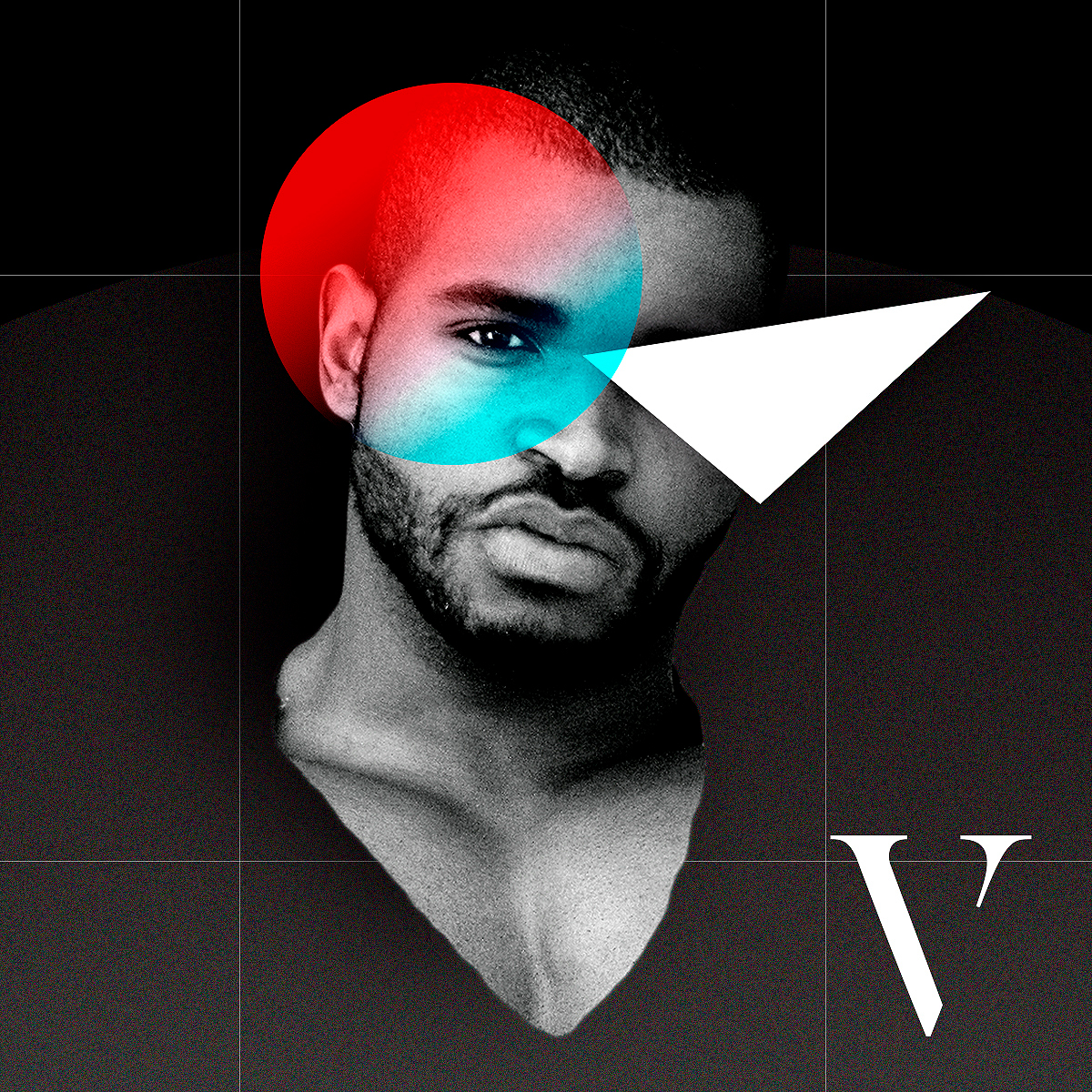# Noturnoo

Members

5

• #### Last visited

• Rank
Newbie
1.## What is the best way to distribute Pixi.Container in different Divs (html elements)?

Thanks a lot for responding, instantiating multiple app, can it impact performance? Well I'll use Pixi to manipulate images, and some of them will be in FullScreen.
2.## What is the best way to distribute Pixi.Container in different Divs (html elements)?

Example, I have 6 divs inside my body, and wanted to add a container for each div: eg.: <div id = "one"> Pixi.Container 1 </ div>, <div id = "two"> Pixi.Container 2</ div>, ..... <div id = "six"> Pixi.Container 6 </ div> and so on, is there a way to do this without having to instantiate several Pixi App?
3. Still did not work Sprite simply loses position app.ticker.add(function(delta) { var speed = 0.8; var delta = 1; var dt = speed; // fixed step var dt = 1.0 - Math.exp(1.0 - dt, delta); // if you have a delta time in frame. const position = displacementSprite.position; const target = app.renderer.plugins.interaction.mouse.global; console.log(target) if (Math.abs(position.x - target.x) + Math.abs(position.y -target.y) < 1) { position.copy(target); } else { position.x = position.x + (target.x - position.x) * dt; position.y = position.y + (target.y - position.y) * dt; } });
4. Hello Ivan, first of all thanks for helping, I really am beginner and I do not know what I should do, I added this at the end of the code, and it did not work: app.ticker.add(function(delta) { var speed = 0.1; var dt = speed; // fixed step var dt = 1.0 - Math.exp(1.0 - dt, delta); // if you have a delta time in frame. const position = circ.position; const target = app.plugins.interaction.mouse.global; if (Math.abs(position.x - target.x) + Math.abs(position.y -target.y) < 1) { position.copy(target); } else { position.x = position.x + (target.x - position.x) * dt; position.y = position.y + (target.y - position.y) * dt; } }); that was it?
5. Hi, Pixrs I'm new to PixiJS and would like to ask a question about how to do a delay on mouse move using Pixi (on the element that has the Displacement filter), I removed a part of the example code that is on the official PixiJS website. I created a div (#follow) to show how effect I wish. Anyway, I would like the element to follow the mouse in a smooth way. Code is in Codepen: Thanks